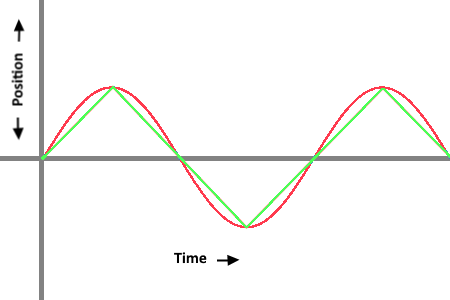# [TOMAJA Micro Video Tutorial] Simple Sinusoidal Function Explanation on Realistic Animation as Example

Hi there!

after reading this discussion about the sinusoidal function using for different effects (breathing, shaking , wave production, floating , movements, animated objects, etc.) I decided to try to explain it in a simple picture and video (gif) form.

Here is the example expression for a moving part in the X Axis (X Position).

(160 + ( 120 * ( sin ( #DWE# * 2 * pi ) ) ) )

And here is a kind of graphic description of this formula.

The original speed (2*pi) in the facer creator could be seen at the following gif picture.

If somebody is more interested to the mathematical explanation, you could find a great explanation here and here .

Please let me know if youthis kind of TOMAJA Micro TutorialsThanks.

16 Likes

This visual aid is awesome. Really clear as opposed to my dense, wordy attempt at explanationYour description, however, has also more information…Putting this here in case someone is looking for a similar alternative with slightly different behavior:

Linear Function, Cycled Motion, but with the same parameters
(where you see 160 that is the “start point”, where you see 120 that is the “distance” and where you see 2*#DWE# that is the “speed”. As you can see, the linear version requires all these inputs more than once)

Original: (160 + ( 120 * ( sin ( #DWE# * 2 * pi ) ) ) )
vs
Linear: \$ (round( floor( 2 * #DWE#+.5 ) / 2 )) = (floor( (2 * #DWE#+.5 ) / 2 )) ? 160 + 120 * (-1 + 2 * ( ( 2 * #DWE#+.5 ) - (floor( 2 * #DWE#+.5 )) ) ) : 160 - 120 * (-1 + 2 * ( ( 2 * #DWE#+.5 ) - (floor( 2 * #DWE#+.5 )) ) ) \$

(the +.5 to each #DWE# starts the cycle from the middle rather than from the left side)

Waveform comparison:Movement comparison:

4 Likes